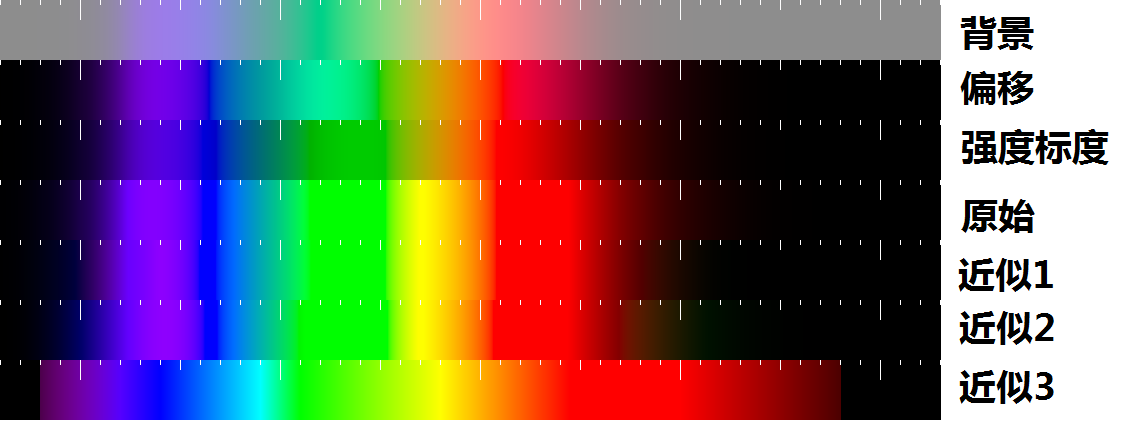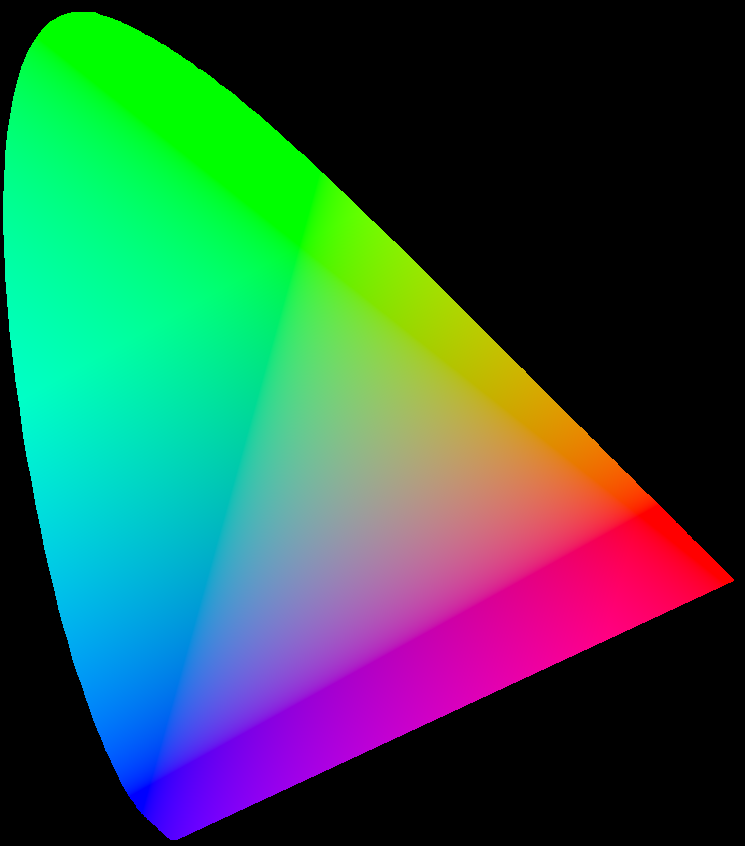# 从光谱到颜色

## 2014-12-08 11:02:23

$\alg X &= k \int S(\l) R(\l) \bar x(\l) d\l \\ Y &= k \int S(\l) R(\l) \bar y(\l) d\l \\ Z &= k \int S(\l) R(\l) \bar z(\l) d\l \\ k &= {100 \over \int S(\l) R(\l) \bar y(\l) d\l} \\ \ealg$

$x={X \over X+Y+Z}, y={Y \over X+Y+Z}$### 参考资料

◆本文地址: , 转载请注明◆
◆评论问题: https://jerkwin.herokuapp.com/category/3/博客, 欢迎留言◆访问人次(2015年7月 9日起): | 最后更新: 2023-08-17 03:23:12 UTC | 版权所有 © 2008 - 2023 Jerkwin